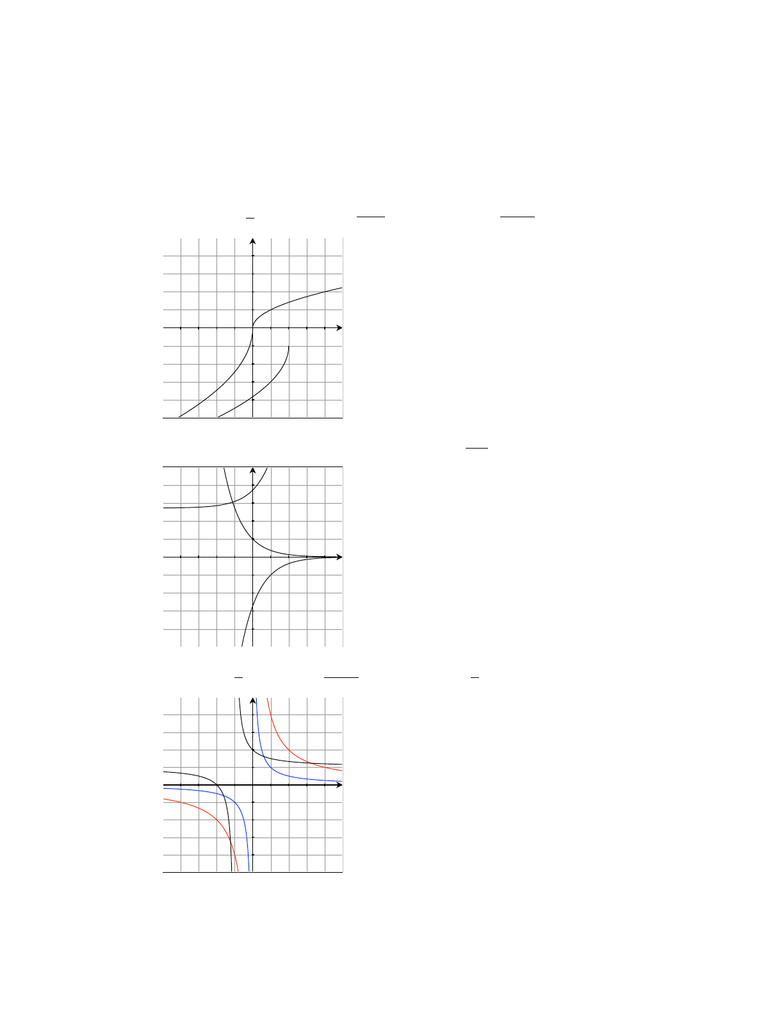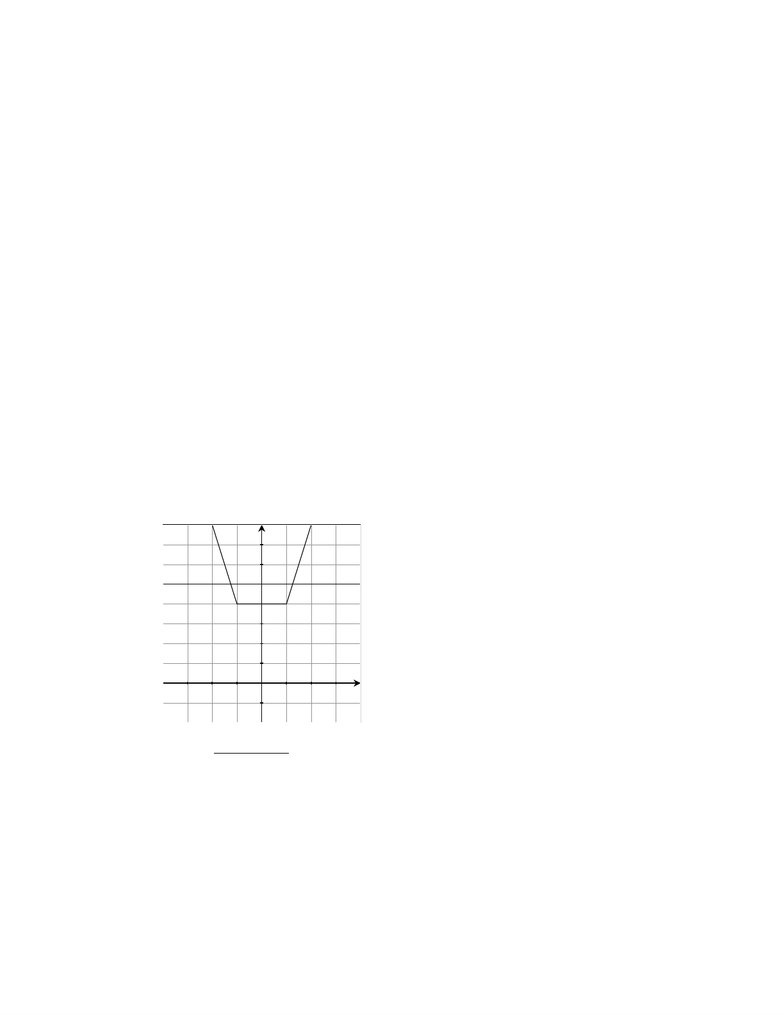Study Guides (390,000)
CA (150,000)
Western (10,000)
MATH (100)
Quiz

# Mathematics 0110A/B Study Guide - Quiz Guide: Farad

Department
Mathematics
Course Code
MATH 0110A/B
Professor
Chris Brandl
Study Guide
Quiz

This preview shows pages 1-2. to view the full 7 pages of the document.MATH 127 Fall 2012
Assignment 2 Solutions
1. For each part, sketch all of the given functions on the same set of axes.
(a) (i) f(x) = x(ii) g(x) = 6x(iii) h(x) = 22x1
-5 -4 -3 -2 -1 0 12345
-5
-4
-3
-2
-1
1
2
3
4
5
y=f(x)
y=g(x)
y=h(x)
(b) (i) f(x) = ex(ii) g(x) = ex+1 (iii) h(x) = 1
ex+e
-5 -4 -3 -2 -1 0 12345
-5
-4
-3
-2
-1
1
2
3
4
5
y=f(x)
y=g(x)
y=h(x)
(c) (i) f(x) = 1
x(ii) g(x) = 1
x+ 1 + 1 (iii) h(x) = 4
x
-5 -4 -3 -2 -1 0 12345
-5
-4
-3
-2
-1
1
2
3
4
5
y=g(x)
y=h(x)
y=f(x)
1

Only pages 1-2 are available for preview. Some parts have been intentionally blurred.2. Sketch each function.
(a) f(x) = |x+ 1|+|x1|(hint: this can be expressed as a piecewise function, show
the work in getting this piecewise function)
We have that x+ 1 0 when x≥ −1 and that x10 when x1. We now
consider the three intervals x < 1, 1x < 1, and x1.
For the ﬁrst interval x < 1 we have that x+ 1 <0 and x1<0 so we get that
f(x) = |x+ 1|+|x1|=(x+ 1) (x1) = x1x+ 1 = 2x.
In the second interval 1x < 1 we have that x+ 1 0 and x1<0 so we
get that f(x) = |x+ 1|+|x1|= (x+ 1) (x1) = x+ 1 x+ 1 = 2.
In the third interval x1 we have that x+ 1 0 and x10 so we get that
f(x) = |x+ 1|+|x1|= (x+ 1) + (x1) = x+1+x1 = 2x.
Thus we have that f(x) = |x+ 1|+|x1|=
2xif x < 1
2 if 1x < 1
2xif x1
-4 -3 -2 -1 01234
-1
-0.5
0.5
1
1.5
2
2.5
3
3.5
4
(b) f(x) = 1
x24x+ 6 (hint: ﬁrst look at the graph of x24x+ 6)
First consider the graph of y=x24x+ 6. Completing the square we can re-
write this as y= (x2)2+ 2 so we see that this is a parabola shifted 2 to the
right and 2 up. The graph of y= (x2)2+ 1 goes through the point (2,2) and
approaches +for large negative and positive values of x. From this we see that
2